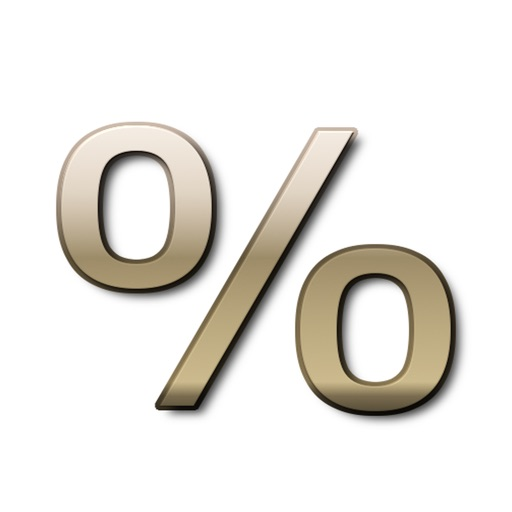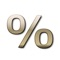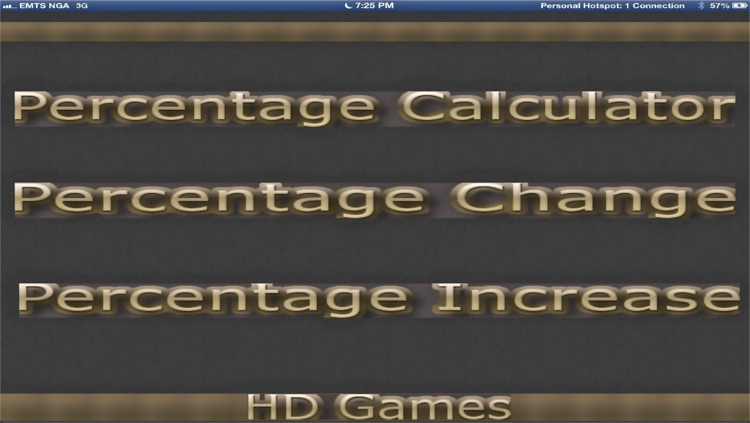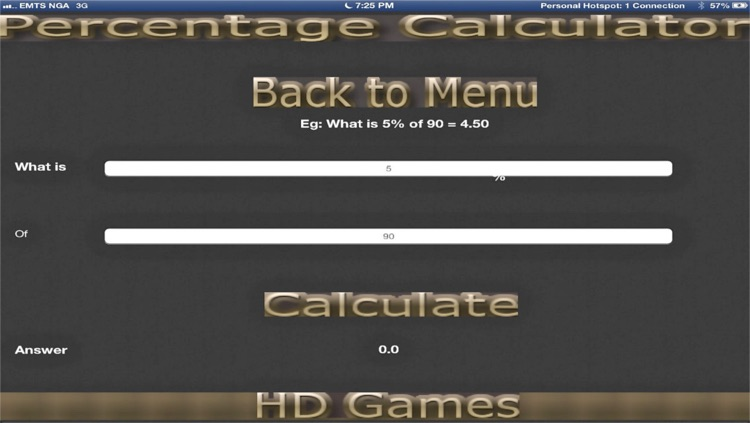## Percentage Calculator is a 3 in one hd app which calculates:# Percentage Calculator 3 in one

by princely EjikemePercentage Calculator is a 3 in one hd app which calculates:### App Details

Version
1.0.1
Rating
(5)
Size
15Mb
Genre
Last updated
December 31, 2013
Release date
November 28, 2013

### Percentage Calculator 3 in one is FREE but there are more add-ons

• \$0.99

Discount calculator

• \$0.99

### App Store Description

Percentage Calculator is a 3 in one hd app which calculates:
1 .Calculate Percents & Percentages
2. Percent Discounts (sale price)
3. Percent Markups (increase by)
4. Percent Margin
5. Percentage Difference (Change)
6.Percentage (what % of) i.e. x is what percentage of y

Percentage calculator is unique in a way that it calculates everything on the fly just by a touch of a button. it lets you find out any part of the equasion, for example in "5% of 40 is 2" you will enter any two of these three numbers and third one would be calculated for you. And that is what makes this percentage calculator stand out in the crowd.
Percentage calculator can be used in many real-life situations as calculator in
1. school (mathematics, statistics, algebra) calculator